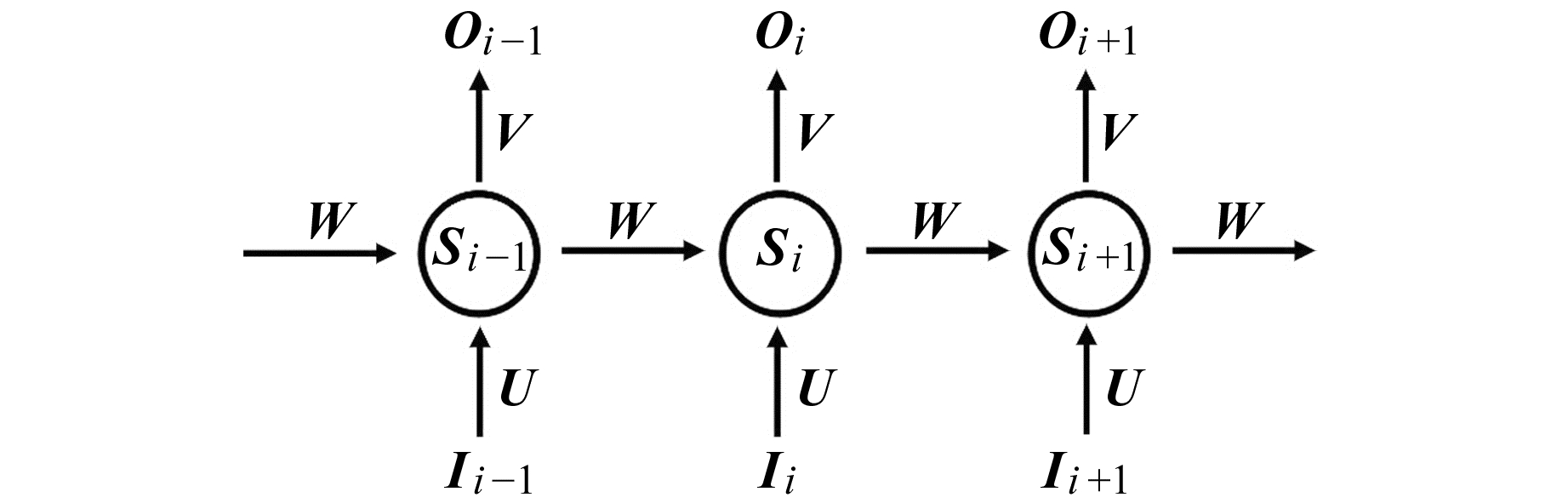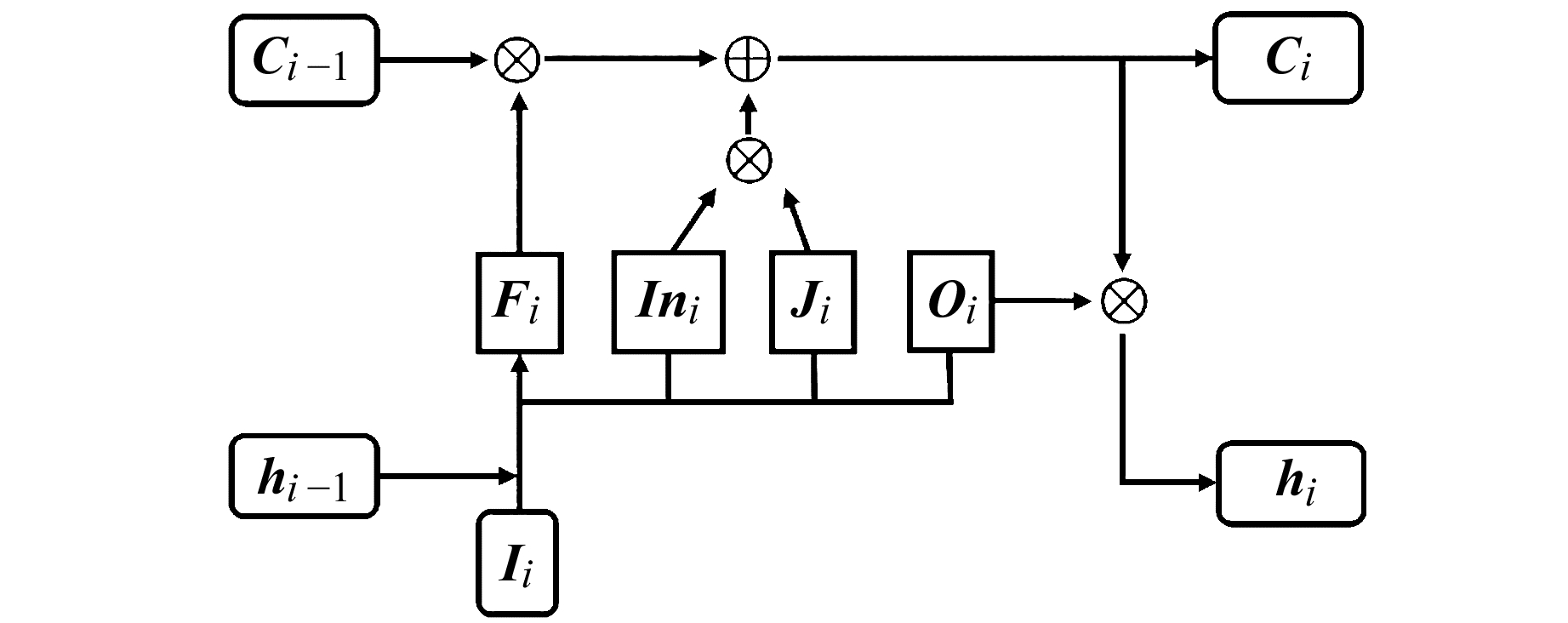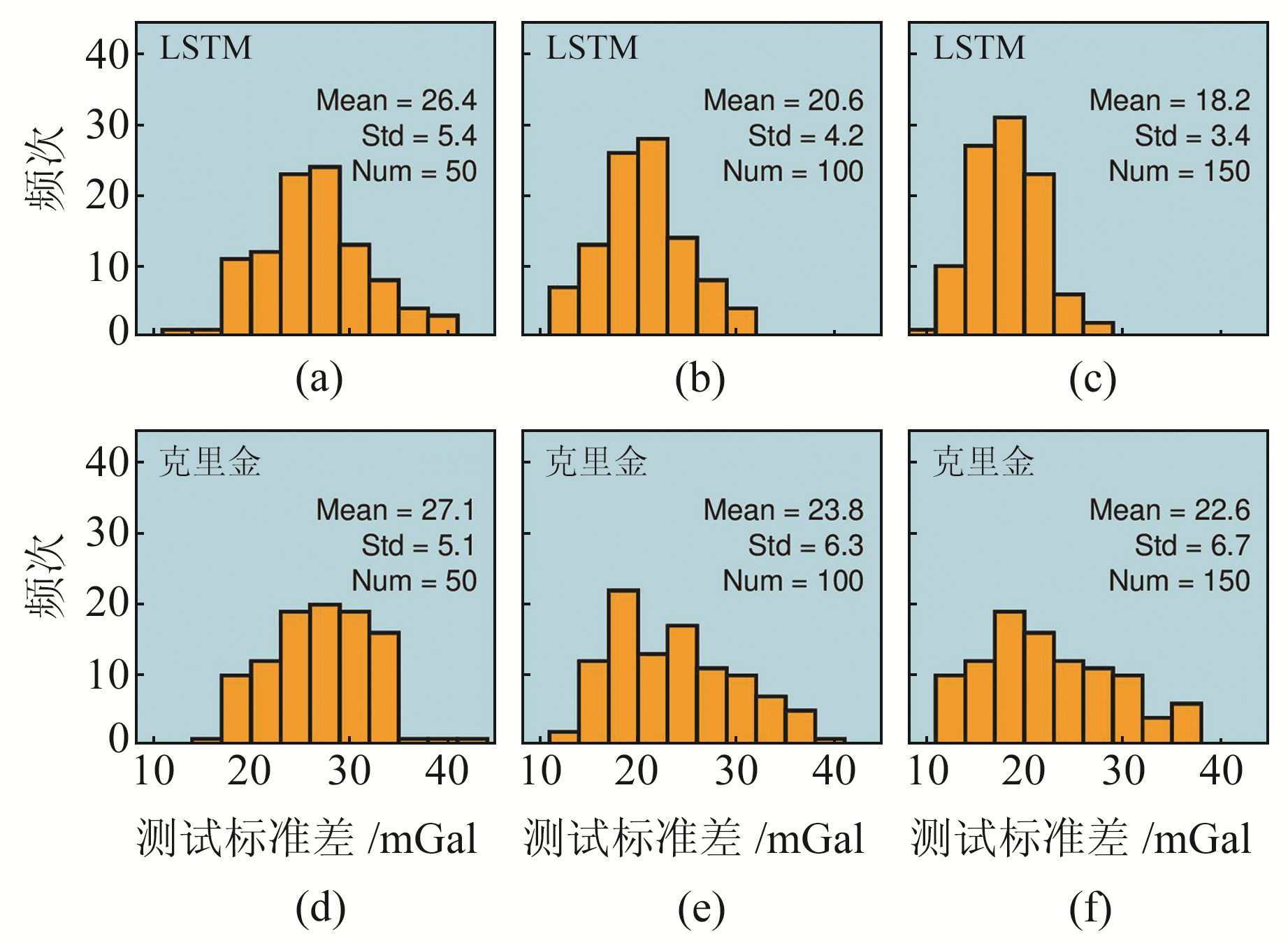﻿ 基于循环神经网络的重力异常数据推估研究文章快速检索 高级检索
 大地测量与地球动力学2021, Vol. 41Issue (3): 234-237  DOI: 10.14075/j.jgg.2021.03.003### 引用本文SHE Yawen, FU Guangyu. Estimation of Gravity Anomaly Data Based on Recurrent Neural Network[J]. Journal of Geodesy and Geodynamics, 2021, 41(3): 234-237.### Foundation support

National Key Research and Development Program of China, No.2018YFC1503503, 2018YFC1503704; National Natural Science Foundation of China, No.41874003; Special Fund for Basic Scientific Research of Institute of Earthquake Forecasting, CEA, No.2020IEF0502, 2020IEF0708.

### Corresponding author

FU Guangyu, PhD, researcher, majors in seismic dislocation deformation and gravity, E-mail: fugy@ief.ac.cn.

### About the first author

SHE Yawen, PhD, majors in gravity observation and interpretation, E-mail: sheyawen@outlook.com.

### 文章历史

1. 南京大学地球科学与工程学院，南京市仙林大道163号，210023;
2. 中国地震局地震预测研究所地震预测重点实验室，北京市复兴路63号，100036

1 自由空气重力异常数据图 1 鄂尔多斯西南缘自由空气重力异常场 Fig. 1 Free-air gravity anomaly field in the southwestern margin of Ordos
2 长短期记忆循环神经网络 2.1 循环神经网络图 2 循环神经网络结构 Fig. 2 Structure of recurrent neural network
 $\boldsymbol{O}_{i}=g\left(\boldsymbol{V} \boldsymbol{S}_{i}\right)$ (1)
 $\boldsymbol{S}_{i}=f\left(\boldsymbol{U} \boldsymbol{I}_{i}+\boldsymbol{W} \boldsymbol{S}_{i-1}\right)$ (2)

 $\begin{array}{c} \boldsymbol{O}_{i}=g\left(\boldsymbol{V} f\left(\boldsymbol{U} \boldsymbol{I}_{i}+\boldsymbol{W} f\left(\boldsymbol{U} \boldsymbol{I}_{i-1}+\boldsymbol{W} f\left(\boldsymbol{U} \boldsymbol{I}_{i-2}+\right.\right.\right.\right. \\ \left.\left.\left.\boldsymbol{W} f\left(\boldsymbol{U}\boldsymbol{I}_{i-3}+\boldsymbol{\cdots}\right)\right)\right)\right) \end{array}$ (3)

2.2 长短期记忆神经元

LSTM将RNN中Si替换为2个输出值进行处理，即神经元的当前状态值Ci和输出值hiCi可保存序列数据的长期状态，通过遗忘门(Fi)、输入门(Ii)和输出门(Oi)进行控制。图 3为LSTM神经元结构图，其中各变量之间的数学关系见式(4)：图 3 长短期记忆神经网络结构 Fig. 3 Structure of long short-term memory
 $\begin{array}{l} \boldsymbol{I} \boldsymbol{n}_{i}=\tanh \left(\boldsymbol{W}_{x I n} \boldsymbol{I}_{i}+\boldsymbol{W}_{h I n} \boldsymbol{h}_{i-1}+b_{i}\right) \\ \boldsymbol{J}_{i}=\operatorname{sigm}\left(\boldsymbol{W}_{x j} \boldsymbol{I}_{i}+\boldsymbol{W}_{h j} \boldsymbol{h}_{i-1}+b_{j}\right) \\ \boldsymbol{F}_{i}=\operatorname{sigm}\left(\boldsymbol{W}_{x f} \boldsymbol{I}_{i}+\boldsymbol{W}_{h f} \boldsymbol{h}_{i-1}+b_{f}\right) \\ \boldsymbol{O}_{i}=\tanh \left(\boldsymbol{W}_{x o} \boldsymbol{I}_{i}+\boldsymbol{W}_{h o} \boldsymbol{h}_{i-1}+b_{o}\right) \\ \boldsymbol{C}_{i}=\boldsymbol{C}_{i-1} \odot \boldsymbol{F}_{i}+\boldsymbol{I}_{i} \odot \boldsymbol{J}_{i} \\ \boldsymbol{h}_{i}=\tanh \left(\boldsymbol{C}_{i}\right) \odot \boldsymbol{O}_{i} \end{array}$ (4)

3 不同推估方法对比分析图 4 基于观测数据的不同推估方法对比 Fig. 4 Comparison of different estimated methods based on observation data图 5 基于不同推估方法的鄂尔多斯西南缘自由空气重力异常场 Fig. 5 Free-air gravity anomaly field in the southwestern margin of Ordos based on different estimation methods
4 结语

  Fu G Y, Gao S H, Freymueller J T, et al. Bouguer Gravity Anomaly and Isostasy at Western Sichuan Basin Revealed by New Gravity Surveys[J]. Journal of Geophysical Research: Solid Earth, 2014, 119(4): 3925-3938 DOI:10.1002/2014JB011033 (0)  She Y W, Fu G Y, Wang Z H, et al. Gravity Anomalies and Lithospheric Flexure around the Longmenshan Deduced from Combinations of in Situ Observations and EGM2008 Data[J]. Earth, Planets and Space, 2016, 68(1): 163 DOI:10.1186/s40623-016-0537-7 (0)  Fu G Y, She Y W. Gravity Anomalies and Isostasy Deduced from New Dense Gravimetry around the Tsangpo Gorge, Tibet[J]. Geophysical Research Letters, 2017, 44(20): 10233-10239 DOI:10.1002/2017GL075290 (0)  申重阳, 杨光亮, 谈洪波, 等. 维西-贵阳剖面重力异常与地壳密度结构特征[J]. 地球物理学报, 2015, 58(11): 3952-3964 (Shen Chongyang, Yang Guangliang, Tan Hongbo, et al. Gravity Anomalies and Crustal Density Structure Characteristics of Profile Weixi-Guiyang[J]. Chinese Journal of Geophysics, 2015, 58(11): 3952-3964 DOI:10.3969/j.issn.0253-4975.2015.09.077) (0)  周志华. 机器学习[M]. 北京: 清华大学出版社, 2016 (Zhou Zhihua. Machine Learning[M]. Beijing: Tsinghua University Press, 2016) (0)  Jozefowicz R, Zaremba W, Sutskever I. An Empirical Exploration of Recurrent Network Architectures[C]. International Conference on Machine Learning, Lille, 2015 (0)  Wang Z Y, Fu G Y, She Y W. Crustal Density Structure, Lithosphere Flexure Mechanism, and Isostatic State throughout the Qinling Orogen Revealed by in Situ Dense Gravity Observations[J]. Journal of Geophysical Research: Solid Earth, 2018, 123(11): 10026-10039 DOI:10.1029/2018JB016117 (0)  刘冬至, 李辉, 刘绍府. 重力测量资料的处理系统——LGADJ[A]//国家地震局科技监测司. 地震预报方法实用化研究文集: 形变、重力、应变专辑[M]. 北京: 地震出版社, 1991 (Liu Dongzhi, Li Hui, Liu Shaofu. A Data Processing System of Gravity Surveying—LGADJ[A]//Science and Technology Monitoring Department of CEA.Practical Research Collection of Earthquake Prediction Methods(Special Issue on Deformation, Gravity and Strain Measurement)[M]. Beijing: Seismological Press, 1991) (0)  Bengio Y, Simard P, Frasconi P. Learning Long-Term Dependencies with Gradient Descent Is Difficult[J]. IEEE Transactions on Neural Networks, 1994, 5(2): 157-166 DOI:10.1109/72.279181 (0)  Hochreiter S, Schmidhuber J. Long Short-Term Memory[J]. Neural Computation, 1997, 9(8): 1735-1780 DOI:10.1162/neco.1997.9.8.1735 (0)  Kitanidis P K. Introduction to Geostatistics: Applications in Hydrogeology[M]. Cambridge: Cambridge University Press, 1997 (0)  Förste C, Bruinsma S L, Abrikosov O, et al. EIGEN-6C4-The Latest Combined Global Gravity Field Model Including GOCE Data up to Degree and Order 1949 of GFZ Potsdam and GRGS Toulouse[C]. EGU General Assembly Conference, Vienna, 2014 (0)  Amante C, Eakins B W. ETOPO11 Arc-Minute Global Relief Model: Procedures, Data Sources and Analysis[Z]. NOAA Technical Memorandum NESDIS NGDC-24, 2009 (0)  任强强, 王跃钢, 腾红磊, 等. 基于小波神经网络的盲区重力数据预测[J]. 大地测量与地球动力学, 2016, 36(4): 359-363 (Ren Qiangqiang, Wang Yuegang, Teng Honglei, et al. The Gravity Data Forecast of Unmeasurable Zone Based on Wavelet Neural Network[J]. Journal of Geodesy and Geodynamics, 2016, 36(4): 359-363 DOI:10.14075/j.jgg.2016.04.018) (0)
Estimation of Gravity Anomaly Data Based on Recurrent Neural Network
SHE Yawen1,2FU Guangyu21. School of Earth Sciences and Engineering, Nanjing University, 163 Xianlin Road, Nanjing 210023, China;
2. Key Laboratory of Earthquake Forecasting, Institute of Earthquake Forecasting, CEA, 63 Fuxing Road, Beijing 100036, China
Abstract: We adopt the gravity observation data of the southwestern margin of Ordos to train the long short-term memory recurrent neural network (LSTM). The results show that LSTM can obtain good estimation results based on limited data. Through comparing and analyzing the estimated results of LSTM and ordinary Kriging method based on free-air gravity anomaly data, we find that the estimation ability of neural network is better than ordinary Kriging method, but Kriging method performs better in terms of computing efficiency. Using the free-air gravity anomaly data to estimate the entire area, we show that LSTM method is significantly better than Kriging method. Adding elevation data as a constraint can effectively improve the accuracy of free-air gravity anomaly field estimated by LSTM method.
Key words: long short-term memory recurrent neural network; free-air gravity anomaly; Kriging method